Courses

# Test: General Intelligence & Reasoning- 2

## 50 Questions MCQ Test Mock Test Series for SSC JE Electrical Engineering | Test: General Intelligence & Reasoning- 2

Description
This mock test of Test: General Intelligence & Reasoning- 2 for SSC helps you for every SSC entrance exam. This contains 50 Multiple Choice Questions for SSC Test: General Intelligence & Reasoning- 2 (mcq) to study with solutions a complete question bank. The solved questions answers in this Test: General Intelligence & Reasoning- 2 quiz give you a good mix of easy questions and tough questions. SSC students definitely take this Test: General Intelligence & Reasoning- 2 exercise for a better result in the exam. You can find other Test: General Intelligence & Reasoning- 2 extra questions, long questions & short questions for SSC on EduRev as well by searching above.
QUESTION: 1

### In the following question, select the related word from the given alternatives. College : Dean ∷ Museum : ?

Solution:

Dean is the in charge of the college.

Similarly, Curator is the in charge of the museum.

QUESTION: 2

### Select the related word from the alternatives: Pig : Sty :: Dog : ?

Solution:

Pig is an animal which lives in a sty. Dog lives in a Kennel,

Hence the answer is option Kennel.

QUESTION: 3

### In the following question, select the related word pair from the given alternatives. Football : Outdoor : : ? : ?

Solution:

‘Football’ is an ‘Outdoor’ game. Similarly, ‘Snooker’ is an ‘Indoor’ game.

QUESTION: 4

Select the related number from the given alternatives.

5 : -10 : : -8 : ?

Solution:

The pattern followed is: x : -2x

→ 5 : -2 × 5 = -10

Similarly,

→ -8 : -2 × (-8) = 16

Hence, the correct answer is 16

QUESTION: 5

In each of the following questions, select the related number from the given alternatives.

5 : 24 : : 23 : ?

Solution:

In 5 : 24
→ 5 × 5 = 25 – 1 = 24
Similarly;
→ 23 × 5 = 115 – 1 = 114
Hence, 5 : 24 : : 23 : 114

QUESTION: 6

In the following question, select the related numbers from the given alternatives.

J : 90 ∷ N : ?

Solution:

With reference to English alphabets,

J = 10

J2 – J = 102 – 10 = 100 – 10 = 90.

Similarly,

N = 14

N2 – N = 142 – 14 = 196 – 14 = 182.

QUESTION: 7

Select the related letters from the given alternatives.

ZWU : TQO : : LIG : ?

Solution:

In ZWU : TQO

Z – 3 = W; W – 2 = U

U – 1 = T

T – 3 = Q; Q – 2 = O

Similarly, in LIG : ?

L – 3 = I; I – 2 = G

G – 1 = F

F – 3 = C; C – 2 = A

QUESTION: 8

In the following question, select the odd letters from the given alternatives.

ACEG : DFHJ :: QSUW : ?

Solution:Similarly,Hence, QSUW is related to TVXZ.

QUESTION: 9

Select the related letters from the given alternatives.

VTHB : KJDA ∷ ? : CMEI

Solution:

We know that position of alphabet is A = 1; B = 2; C = 3…and so on.

In VTHB : KJDA

VTHB their position in English alphabet. ⇒ V = 22, T = 20, H = 8, B = 2

KJDA their position in English alphabet. ⇒ K = 11, J = 10, D = 4, A = 1

Similarly,

FZJR their position in English alphabet ⇒ F = 6, Z = 26, J = 10, R = 18

CMEItheir position in English alphabet. ⇒ C = 3, M = 13, E = 5, I = 9

Hence, “FZJR” is missing term.

QUESTION: 10

In the following question, select the odd number from the given alternatives.

Solution:

The logic followed is:

Sum of all the digits in the number is not a prime number, except in option 1.

1) 788 → 7 + 8 + 8 = 23, which is a prime number.

2) 654 → 6 + 5 + 4 = 15, which is not a prime number.

3) 984 → 9 + 8 + 4 = 21, which is not a prime number.

4) 824 → 8 + 2 + 4 = 14, which is not a prime number.

Hence, “788” is the odd one among the given alternatives.

QUESTION: 11

Find out the odd number from the given alternatives.

Solution:

The logic followed is:

1) 29 → 52 + 4

2) 85 → 92 + 4

3) 147 → 122 + 3

4) 125 → 112 + 4

Except “147” all other numbers are in the form n2 + 4.

Hence, “147” is the odd one among the given alternatives.

QUESTION: 12

Find the odd number pair from the given alternatives.

Solution:

The two digits of the given number are reversed and its pair is written with the reversed digits.

So 46 - 54 has to be 46 - 64.

Hence pair 46 - 54 is odd one.

QUESTION: 13

In the following question, select the odd word pair from the given alternatives.

Solution:

All are there part of colour except colour - green.

QUESTION: 14

Choose the word which is different from the rest.

Solution:

All except Bear belong to the cat family.

Hence, Bear is different from rest.

QUESTION: 15

In question find the word which is different from the given alternatives.

Solution:

→ Keyboard, Mouse and Monitor are hardware component of computer but MySql is software to store data in database.

QUESTION: 16

In the following question, select the odd letter/letters from the given alternatives.

Solution:

The pattern followed here is,

1) T – 3 = Q, Q – 3 = N, N – 3 = K

2) U – 3 = R, R – 3 = O (N), O (N) – 3 = L (M)

3) V – 3 = S, S – 3 = P, P – 3 = M

4) L – 3 = I, I – 3 = F, F – 3 = C

Hence, the odd one is ‘URNM’.

QUESTION: 17

In the following question, select the odd letters from the given alternatives.

Solution:

1) M + 7 = T;

2) C + 7 = J;

3) S + 7 = Z;

4) F + 8 = N

Hence, FN is odd one out.

QUESTION: 18

In the following question, select the odd word pair from the given alternatives.

Solution:

In all the pairs, the 1st word is an Antonym of 2nd word i.e.

Up - Down → Up is an antonym of Down.

Increase - Decrease → Increase is an antonym of Decrease.

Cry - Laugh → Cry is an antonym of Laugh.

But in, Walking - Running → Walking is not an antonym of Running.

Thus, Walking - Running is the odd pair.

QUESTION: 19

A series is given, with one term missing. Choose the correct alternative from the given ones that will complete the series.

8, 10, 30, 32, 52, 54, 74, 76, 96, ?

Solution:

The given series is,

8 + (2) → 10 + (20) → 30 + (2) → 32 + (20) → 52 + (2) → 54 + (20) → 74 + (2) → 76 + (20) → 96 + (2) → 98

QUESTION: 20

Complete the following number series.

1, 28, 92, 217, 433, ?

Solution:

1 + 33 = 28,

28 + 43 = 92,

92 + 53 = 217,

217+ 63 = 433,

433 + 73 = 776

Hence the question mark is replaced by 776.

QUESTION: 21

In the following question, choose the correct alternative from the given ones that will complete the series:

21, 24, 32, 45, 63, ?

Solution:

The relation among given numbers is as shown below,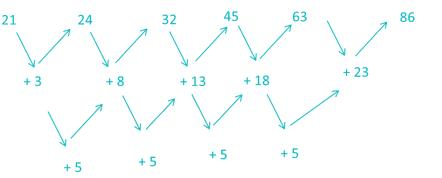Hence, 86 will complete the series.

QUESTION: 22

A series is given with one term missing. Select the correct alternative from the given ones that will complete the series.

LMK, FGE, ZAY, TUS, ?

Solution:

The pattern followed here is,

L - 6 = F; F - 6 = Z; Z - 6 = T; T - 6 = N

M - 6 = G; G - 6 = A; A - 6 = U; U - 6 = O

K - 6 = E; E - 6 = Y; Y - 6 = S; S - 6 = M

Hence, NOM is the correct answer.

QUESTION: 23

Which of the following terms follows the trend of the given list?

aAAAAAA, AaAAAAA, AAaAAAA, AAAaAAA, AAAAaAA, _________.

Solution:

According to the sequence, ‘a’ is shifting one position ahead of its next position at every step. Thus next term would be ‘AAAAAaA’.

QUESTION: 24

Complete the series.

AZBC, BYCD, CXDE, ?

Solution: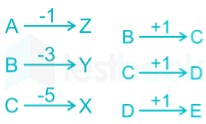Similarly,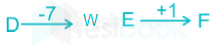Hence, the correct answer is DWEF.

QUESTION: 25

If PROMISE is coded as OPRSIME, then FRIENDS is coded as

Solution:

Here in PROMISE;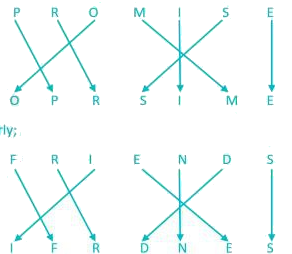QUESTION: 26

In a certain code language,

'mxy das zci' means 'good little frock'

'Jmx cos zci' means 'girl behaves good'

'das ajp cos' means 'little girl fell'

Which word in that language stands for 'frock'?

Solution: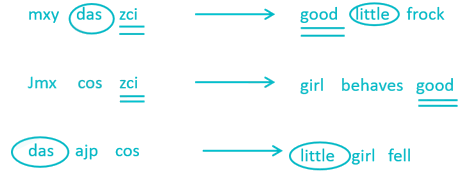In the first and second statements, the common code word is ‘zci’ and the common word is

‘good’.

So, ‘zci’ stands for ‘good’.

In the first and third statements, the common code word is ‘das’ and common word is ‘little’.

So, ‘das’ stands for ‘little’.

Thus, in first statement ‘mxy’ stands for ‘frock’.

QUESTION: 27

Rasik walked 20 m towards north. Then he turned right and walks 30 m. Then he turns right and walks 35 m. Then he turns left and walks 15 m. Finally he turns left and walks 15 m. In which direction and how many metres is he from the starting position?

Solution:

From the information given above we can trace a direction diagram,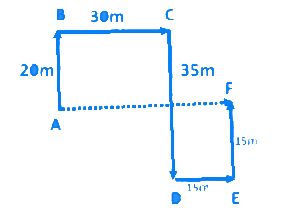Required distance = AF = BC + DE = 30 + 15 = 45 m

As per above diagram F is in east direction with respect to A.

Hence, 45m, east is correct option.

QUESTION: 28

Richa is standing North of Amar and East of Tia and Soha is east of Amar and North - East of Monu and Sonu is South Tia and South- west of Amar. Monu is in which direction from Tia ?

Solution:

It is clear from the diagram that Monu is in South – east direction from Tia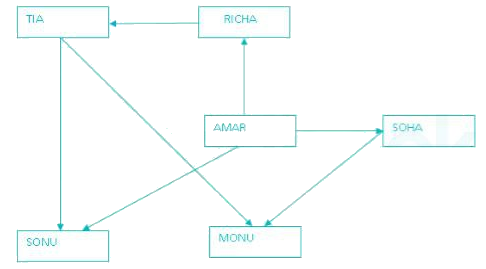QUESTION: 29

Select the missing number from the given responses.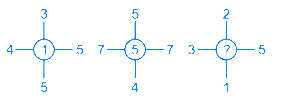Solution:

The central number is found by first finding the sum of numbers opposite to each other and then finding the difference between the resulting sums.

In the first case it is (4 + 5) – (3 + 5) = 1

Similarly, (7 + 7) – (5 + 4) = 5

So, (3 + 5) – (1 + 2) = 5

Hence the missing number is 5.

QUESTION: 30

Which of the following words will come third if arranged according to the English dictionary?

Solution:

The correct order will be:

Prawn → Prayer → Prick → Pride

Thus the third word is Prick.

QUESTION: 31

A is 2 years older to B. B is 5 years younger to C. C is 3 years older to D. D is 6 years younger to E. Who is the eldest?

Solution:

According to the question the relation between the ages of A, B, C, D and E are

A = B + 2

B = C - 5

C = D + 3

D = E - 6

Let the age of E be 10 years

D = 10 - 6 = 4 years

C = D + 3 = 4 + 3 = 7 years

B = C - 5 = 7 - 5 = 2 years

A = B + 2 = 2 + 2 = 4 years

Hence, E is the eldest.

QUESTION: 32

Select the set of symbols which can be fitted correctly in the equation.

8___4___2___6___3 = 32

Solution:

1) ×, –, + , ÷ ⇒ 8 × 4 – 2 + 6 ÷ 3 = 32 – 2 + 2 = 32 (True)

2) + , ×, ÷, – ⇒ 8 + 4 × 2 ÷ 6 – 3 = 8 – 4/3 – 3 ≠ 32 (False)

3) + , + , ×, – ⇒ 8 + 4 + 2 × 6 – 3 = 12 + 12 – 2 ≠ 32 (False)

4) –, x, ÷, + ⇒ 8 – 4 × 2 + 6 ÷ 3 = 8 – 8 + 2 ≠ 32 (False)

Hence, “×, –, + , ÷” give correct equation.

QUESTION: 33

If "–" denotes "divided by", "+" denotes "subtracted from", "×" denotes "added to" and "÷" denotes "multiplied by", then 4 ÷ 16 × 5 + 4 – 2 = ?

Solution: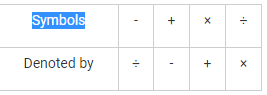Given expression: 4 ÷ 16 × 5 + 4 – 2

After changing: 4 × 16 + 5 - 4 ÷ 2

= 64 + 5 – 2

= 67

Hence, 67 is the correct answer.

QUESTION: 34

F and G are brothers. P and Q are sisters. F’s son is Q’s brother. How G related to P?

Solution:

Table is drawn describing symbols and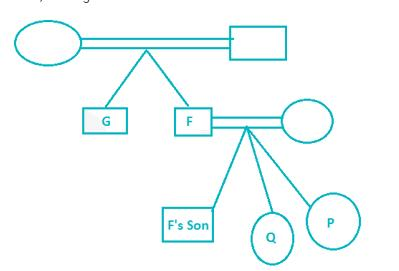their meanings:

F’s son is Q’s brother implies that P and Q are F’s daughters, making P the niece of G.

Hence, G is uncle of P.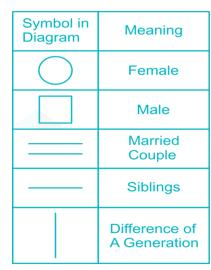Now, solving the statements:

QUESTION: 35

Arrange the following words in the sequence in which they occur in dictionary:

1. Brook,

2. Bandit,

3. Boisterous,

4. Baffle,

5. Bright

Solution:

Clearly, the correct alphabetical order to the given words is baffle,

bandit, boisterous, bright, brook,

Thus, the correct sequence is 4, 2, 3, 5, 1.

QUESTION: 36

Arrange the words in the alphabetical order and tick the one that comes second

Solution:

The given words can be arrange in the alphabetical order as:

eager, ecstasy, emergency, and explosion

clearly, ecstasy comes second.

QUESTION: 37

Select the word which cannot be formed using the letters of the given word

REJUVENATION

Solution:

JUTE → can be formed → REJUVENATION

NATION → can be formed → REJUVENATION

REVISION → cannot be formed as there is no S and only one I

NATIVE → can be formed → EJUVENATION

QUESTION: 38

Which of the following venn diagrams correctly illustrates the relationship among the classes women,  Teacher,  Mother?

Solution:

All mother are women and some women and mother are teacher .hence below figure best describes the relationship among Mother, Women and Teacher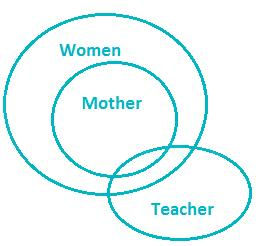QUESTION: 39

In a family, there are six members A, B, C, D, E and F.

A and B are a married couple, A being the male member. D is the only son of C, who is the brother of A. E is the sister of D. B is the daughter-in-law of F, whose husband has died. How is E related to C ?

Solution:

A is a male and married to B. So, A is the husband and B is the wife. C is the brother of A. D is the son of C. E. who is the sister of D will be the daughter of C. B is the daughter-in-law of F whose husband has died means F is the mother of A.

Clearly. E is the daughter of C.

QUESTION: 40

Find the part which represents those actors who are also singers.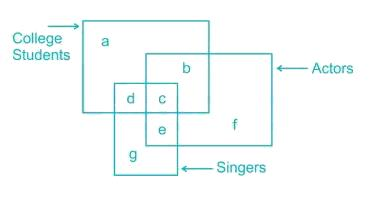Solution: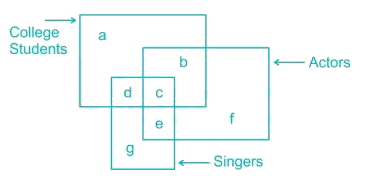QUESTION: 41

Consider the given statement/s to be true and decide which of the given conclusions/assumptions can definitely be drawn from the given statements.

Statements:

I. All elephants are men.

II. All men are socks.

Conclusions:

I. Some socks are elephants.

II. All elephants are socks

Solution:

From the above statements the diagram is as follows:

Conclusions:

I. Some socks are elephants → clearly true.

II. All elephants are socks → clearly true.

Hence, both the conclusions I and II follow.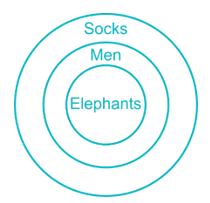QUESTION: 42

Some stools are tables

Conclusions:

I. Some benches are stools

II. Some benches are not chairs is a possibility.

III. All chairs are tables

IV. All tables are stools

Solution: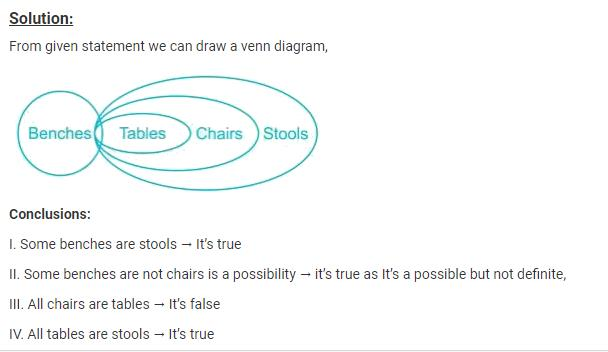QUESTION: 43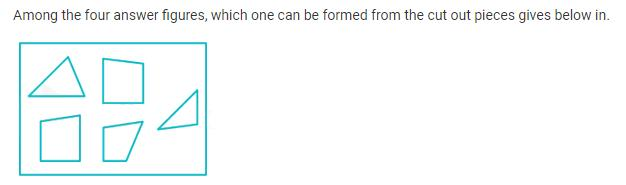Solution: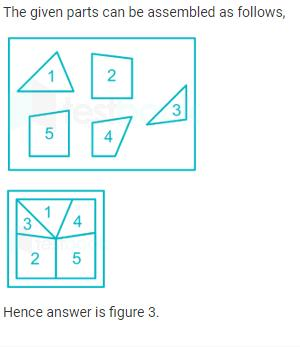QUESTION: 44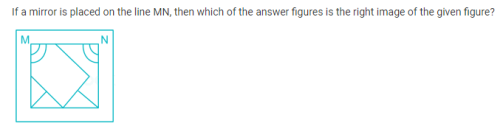Solution: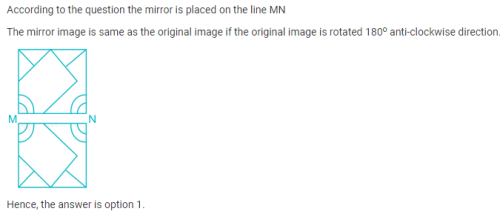QUESTION: 45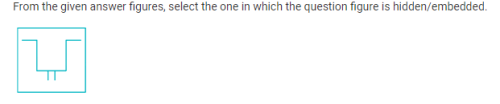Solution:

From the figure we can observe that the figure in the question is hidden in the option A.

In fig B no such pattern is there.

In fig C, the top left part of the pattern is missing.

In fig D, the top right part of the pattern is missing.

So, the correct option is A.

QUESTION: 46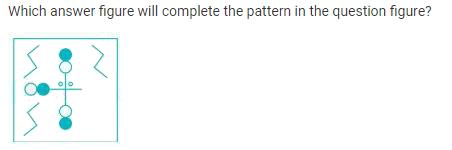Solution: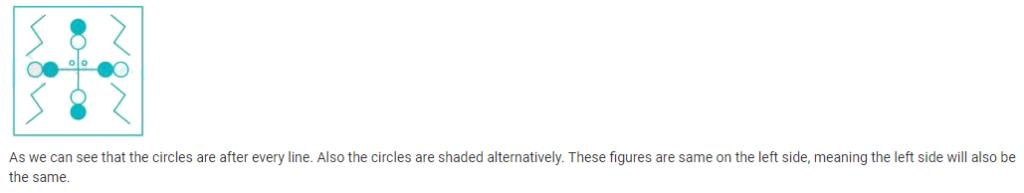QUESTION: 47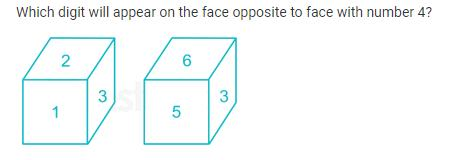Solution: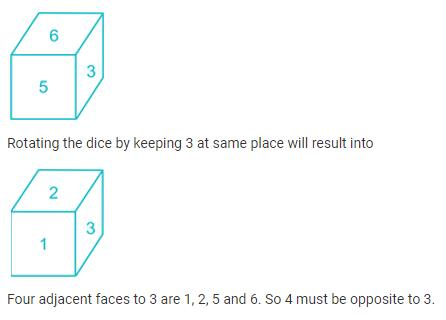QUESTION: 48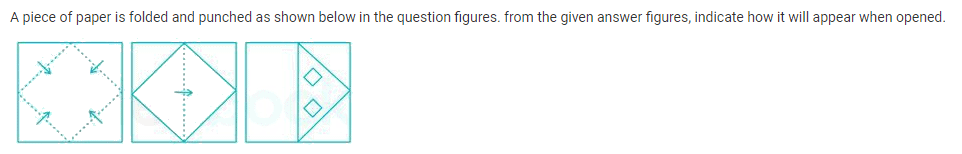Solution: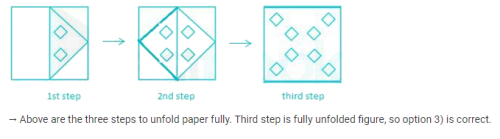QUESTION: 49

In the following question, find the missing number from the given responses.

6    9    8

4    6    6

2    3    4

12  ?    12

Solution:

The relation is as shown below

6 × 4 = 24 ÷ 2 = 12

8 × 6 = 48 ÷ 4 = 12

Similarly,

9 × 6 = 54 ÷ 3 = 18

Hence, the missing number is 18.

QUESTION: 50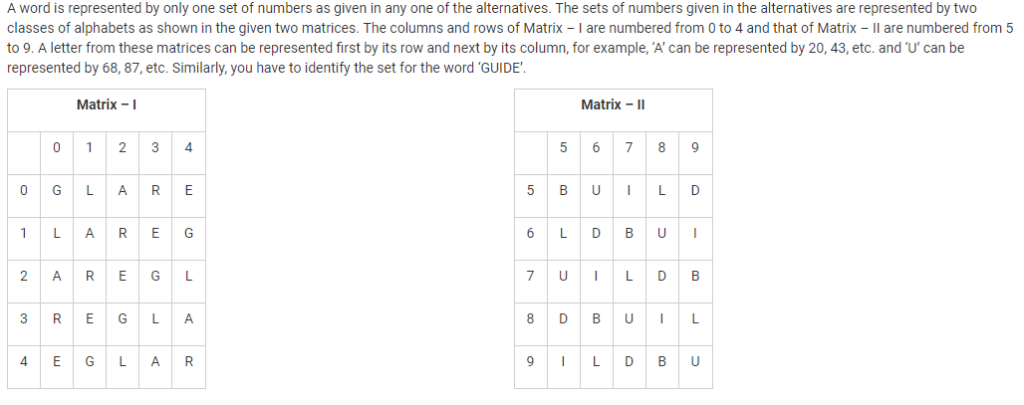Solution:

1) 00, 68, 95, 58, 04 → GUILE

2) 14, 75, 88, 87, 40 → GUIUE

3) 23, 99, 76, 78, 31 → GUIDE

4) 41, 87, 57, 66, 12 → GUIDR

Hence, “23, 99, 76, 78, 31”is the correct set.